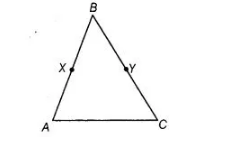`
Question:

In the adjoining figure, if BX = ½ AB, BY = ½ BC and AB = BC, then show that BX = BY.Thinking Process

Apply the Euclid’s axiom, things which are double of the same things are equal to one another.

Solution:

Given, BX = ½AB =>2 BX = AB ,..(i)

BY = ½BC

=» 2 BY = BC …(ii)

and AB = BC …(iii)

On putting the values from Eqs. (i) and (ii) in Eq. (iii), we get

2BX = 2BY

According to Euclid’s axiom, things which are double of the same things are equal to one another.

BX = BY